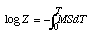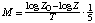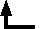## Ludwig Ferdinand Wilhelmy (1812-1864)

### The Law By Which the Action of Acids on Cane Sugar Occurs

excerpt from Poggendorf's Annalen der Physik und Chemie 81, 413-433, 499-526 (1850) [from Henry M. Leicester and Herbert S. Klickstein, eds., A Source Book in Chemistry, 1400-1900 (Cambridge, MA: Harvard, 1952)]

It is known that the action of acids on cane sugar, which rotates the plane of polarized light passing through its solution to the right, converts it into fruit sugar, which rotates the plane to the left. Since now with the aid of a polarization apparatus, the Soleil double plate, readings of how far this change has proceeded can be made with great ease in an instant, it seemed to me to offer the possibility of finding the laws of the process which we are discussing. It is also a problem of interest to establish in this special case. However, this is certainly only one member of a greater series of phenomena which all follow general laws of nature.

...

Under the final assumption, however, the formula which expresses the chemical process can be developed in the following way:

Using the symbols introduced above, dZ is the sugar loss in the time interval dT, and it is assumed that this is determined by the formula

- dZ/dT = MZS
in which M, as before, is the mean value of the infinitely small amount of sugar units which are changed during the time element by the action of the amount of acid present.

The above equation gives by integrationor since, as has already been shown, S is constant, and on the other hand M also is independent of Z and therefore also of T, as will later be shown by experiment,
log Z = -MST + C
For T = 0, Z = Z0, therefore,
log Z0 - log Z = MST, or Z = Z0e-MST.

...

According to the preceding work, I can therefore use the formula developed earlier

log Z0 - log Z = MST

#### A. Influence of Time

A mixture of 10 grams sugar solution (rotation °, 8) and 2 grams nitric acid (specific gravity 1.2042) was placed in the apparatus (tube length 150 millimeters, content 13,850 milligrams distilled water at 15°),and the course of action was observed for a day. The readings gave the values for rotation which follow, and it was noted that the temperature of the room and the liquid at the beginning of the study was 15°, 5. This rose slightly at 1 hr. 30', reached a maximum of 18°, and then at the end of the study fell slowly to 14°, 5. Correspondingly, the difference in the inversion coefficient is calculated at m = 0.3966, decreasing to 0.3846 and rising to 0.404: thus we obtain the values of the expression
(log Z - log Z0)/MS

The convenient half-Briggsian logarithms could be used without disadvantage, since we are concerned only with the ratio of the numbers to each other and not with their absolute values. The figures thus obtained are collected in Table III.

Table III
TRotation[log Z0 - log Z)/MSt
46°, 75 before mixing
8 h.Mixing of acid with sugar solution
8 h. 15'+43°, 7515°5
" 30+41
" 45+38, 25
9 h.+35, 750.0801671
9 h. 15+33, 25
9 h. 30+30, 75
9 h. 45+28, 25
10 h.+260.1661271
10 h. 30+22
11 h.+18, 250.2504869
11 h. 30+15
12 h.+11, 50.3393678
12 h. 30+8, 25
1 h. 30+2, 750.488223818°
2 h. 30-1, 750.585920816°
3 h. 30-4, 50.6628879
4 h. 30-70.747056115°
5 h. 30-8, 750.8173016
6 h. 30-100.875293614°5

As can be seen, the values found during the time which passed are very nearly proportional, from which the correctness of the formula Z = Z0e-MST in relation to time T is established. The small deviation from proportionality is explained by the irregularities of temperature, to which I will return later. A necessary conclusion from the equation, moreover, is that when T = °, can become zero, hence the inversion of the sugar, strictly speaking, is never ended, although due to the smallness of the residue, very soon a further decrease is not noticeable.

#### B. Influence of the Amount of Sugar

It was earlier remarked that the inversion coefficient M is independent of the amount of sugar; this appears from the series of observations already reported. If we calculate M for each time interval by the formulawe get the following values
 M = 0.0204467 . 1/5 (t 15°,5) M = 0.0197215 " M = 0.0204460 " M = 0.0195529 " M = 0.0204748 " M = 0.0214880 " M = 0.0226069 " M = 0.0213903 " M = 0.0210030 " M = 0.0202667 " M = 0.0241737 " M = 0.0240091 " M = 0.0252094 " t 18° (maximum temperature) M = 0.0244242 " M = 0.0192418 " M = 0.0210170 " M = 0.0175614 " M = 0.0142906 " t 14°,5
The slight irregularity in value of M corresponds to the temperature, whose influence will be considered in a special section; thus M remains constant while the amount of sugar in the liquid has fallen from 46,75 to 6,23.

The independence of the value of M of the amount of sugar will be further indicated by a special study. This was found in each of the studies carried out simultaneously and at the same temperatures, in which, besides the amount of acid S and the amount of water W, M by the formula log Z0 - log Z = MST was found constant. The detailed conditions and results are given in Table IV.

Table IV
Z0Anhydrous HNO3WTDM
S
45°0.281 g.7.542 g.13 h.+4°,250.1240
36"""+30.1267
27"""+20.1290
18"""+1,250.1301
9"""+30.1201
Z is, as always, calculated from D by the formula previously mentioned,

Z = Z0 - (Z0-D)/(1+m)

[Namely, that the reaction is independent of acid concentration, according to L&K. --CJG]

[Z = concentration of sugar, S = concentration of acid. --L&K]

Z is found from the observed rotation; D, by the formula Z = Z0 - (Z0 -D)/(1 + m)Back to the list of selected historical papers.Back to the top of Classic Chemistry.